# Bayesian Network in Machine Learning

In this tutorial, we will learn about the Bayesian Network, Bayes Network, and DAG (directed acyclic graph) in machine learning with the help of example. By Bharti Parmar Last updated : April 17, 2023

## What is Bayesian Network?

The Bayesian Network is known as a "Belief Network" or "Student Network" which relies on a directed graph. The Bayesian Network is defined for a rule for finding out the probability of an event given that another event already happened.

```P (One event \ another event)
```

Here, we could isolate the probability of B given A and probability of A Given B and compute for simple probability. It is a very simple and important Bayes theorem problem for calculating the probability.

Diagram: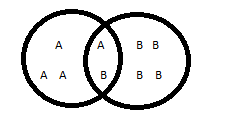```P(A/B) = P(B/A) * P(A) / P(B)
P(B/A) = P(A/B) * P(B) / P(A)
```

## What is Bayes Network?

The Bayes Network is a structure that can be represented as a direct acyclic graph. It allows a compact representation of the distribution from the chain rule of Bayes network. It observes conditional independencies relationships between random variables.

### What is DAG (Directed Acyclic Graph)?

The DAG (Directed Acyclic Graph) is a finite directed graph with no directed cycles.

### Example of Bayes network

Consider the below diagram: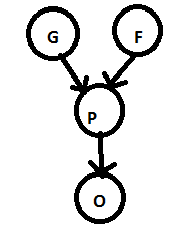There are 4 random variables in the above graph G, F, P, O:

• In Genes (G) 0 is for bad and 1 is for good.
• In bool (F) 0 is for no and 1 for yes.
• Then we check performance in bad, okay and brilliant in the spot or no spot.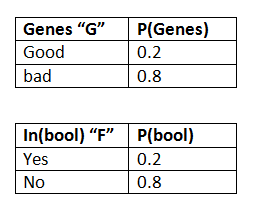The edges in the graph encoded the dependencies in the graph,

• The "Genes" depends on the "good" and "bad and the form in bool "yes" and "no".
• The "Genes" in turn, determines whether the genes are "good" or "bad" with bool form in "yes" or "no".

The direction of arrows tells us about cause-effect relationships — "Performance" affects the "final probability" score.

Finally, let's look at the tables associated with each of the nodes. Formally, these are called conditional probability distributions (CPDs).

### Conditional probability "P"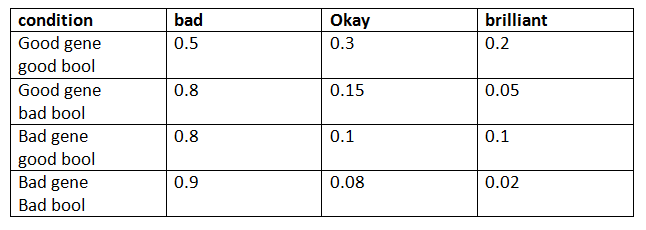The CPDs for "Genes" and "form" are simple, because these variables do not depend on any of the other variables. Basically, the table encodes the probabilities of these variables. As you might have noticed, the values in each of the tables must sum to 1.

The CPD for "O" encodes the conditional probabilities p(prob=O | conditional prob. = P).

The CPD for "P" is easy to understand because it has two parents, the conditional probabilities will be of the form(prob. P | Genes=G, form = F), that is, what is the probability of "prob." being P. Each row now corresponds to a pair of values of "Genes" and "form." Again, the row values add up to 1. An essential requirement for Bayesian networks is that the graph must be a directed acyclic graph (DAG).

### Probability "O"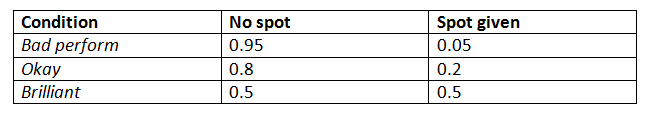### Working

• Each node in the Bayes network will have a CPD associated with it.
• If the node has parents, the associated CPD represents P(value \ Parents value).
• If a node has no parents, the CPD represents P(value), the unconditional probability of the value.

## Conclusion

In this tutorial, we have learned about Bayesian network and Bayes network with example and conditional probability distribution. We will know more about ML in the upcoming article. Have a nice day! Happy learning!